Refer to our Texas Go Math Grade 5 Answer Key Pdf to score good marks in the exams. Test yourself by practicing the problems from Texas Go Math Grade 5 Lesson 2.8 Answer Key Division.

Unlock the Problem

Sean and his crew operate a fishing charter company. They caught a blue marlin and amberjack. The weight of the blue marlin was 12 times as great as the weight of the amberjack. The combined weight of both fish was 1,014 pounds. How much did each fish weigh?

What do I need to find?
I need to find the weight of each fish.

What information am I given?

I know that they caught a total of 1,014 pounds of fish and the weight of the blue marlin was 12 times as great as the weight of the amberjack.

Plan

I can use the strategy of Addition and Substitution and then divide. I can draw and use a strip diagram to write the division problem that helps me find the weight of each fish.

Solve

I will draw one box to show the weight of the amberjack. Then I will draw a strip of 12 boxes of the same size to show the weight of the blue marlin. I can divide the total weight of the two fish by the total number of boxes.Write the quotient in each box. Multiply it by 12 to find the weight of the blue marlin.
So,
The amberjack weighed 78 pounds and the blue marlin weighed 936 pounds.

Try Another Problem

Jason, Murray, and Dana went fishing. Dana caught red snapper. Jason caught a tuna with a weight 3 times as great as the weight of the red snapper. Murray caught a sailfish with a weight 12 times as great as the weight of the red snapper. If the combined weight of the three fish was 208 pounds, how much did the tuna weigh?

What do I need to find?
I need to find the weight of tuna

What information am I given?
I know that Jason, Murray, and Dana went fishing. Dana caught red snapper. Jason caught a tuna with a weight 3 times as great as the weight of the red snapper. Murray caught a sailfish with a weight 12 times as great as the weight of the red snapper and the combined weight of the three fish was 208 pounds

Plan

What is my plan or strategy?
I can use the strategy of Addition and Substitution and then divide

Solve
It is given that
Jason, Murray, and Dana went fishing. Dana caught red snapper. Jason caught a tuna with a weight 3 times as great as the weight of the red snapper. Murray caught a sailfish with a weight 12 times as great as the weight of the red snapper and the combined weight of the three fish was 208 pounds
Now,
Let the weight of the red snapper be: x Pounds
So,
The weight of the fish caught by Dana = x Pounds
The weight of the tuna caught by Jason = 3x Pounds
The weight of the saltfish caught by Murray = 12x Pounds
Now,
According to the combined information,
x + 3x + 12x = 208 Pounds
16x = 208
x = $$\frac{208}{16}$$
= 13 Pounds
So,
The weight of the tuna caught by Jason = 3x
= 3 × 13
= 39 Pounds
So,
The tuna weighed 39 pounds.

Substitute the weight of the tuna in the total weight of the three fishes and check whether the answer is equal to the combined weight of the fishes caught by Dana and Murray

Math Talk
Mathematical Processes

Explain how you could use another strategy to solve this problem.
The other strategy to solve the above problem is:
Step 1:
Step 2:
Plan the strategy how to find the required quantity from the given information
Step 3:
Solve the problem and find the required quantity

Share and Show

Question 1.
Paula caught a tarpon with a weight that was 10 times as great as the weight of a permit fish she caught. The total weight of the two fish was 132 pounds. How much did each fish weigh?
First, draw one box to represent the weight of the permit fish and ten boxes to represent the weight of the tarpon.
Next, divide the total weight of the two fish by the total number of boxes you drew. Place the quotient in each box.
Last, find the weight of each fish.
The permit fish weighed __________ pounds. The tarpon weighed ___________ pounds.
It is given that
Paula caught a tarpon with a weight that was 10 times as great as the weight of a permit fish she caught. The total weight of the two fish was 132 pounds
Now,
Let the weight of the permit fish be: x pounds
So,
The weight of the tarpon is: 10x Pounds
Now,
According to the given information,
x + 10x = 132 Pounds
11x = 132 pounds
x = $$\frac{132}{11}$$
Now,So,
The weight of the permit fish is: 12 pounds
The weight of the tarpon is: 120 pounds
Hence, from the above,
We can conclude that
The weight of the permit fish is: 12 pounds
The weight of the tarpon is: 120 pounds

Question 2.
What if The weight of the tarpon was 11 times the weight of the permit fish, and the total weight of the two fish was 132 pounds? How much would each fish weigh?
permit fish: __________ pounds
tarpon: __________pounds
It is given that
The weight of the tarpon was 11 times the weight of the permit fish, and the total weight of the two fish was 132 pounds
Now,
Let the weight of the permit fish be: x pounds
So,
The weight of the tarpon is: 11x pounds
So,
According to the given information,
11x + x = 132 pounds
12x = 132 pounds
x = $$\frac{132}{12}$$
Now,So,
The weight of the permit fish is: 11 pounds
The weight of the tarpon is: 121 pounds
Hence, from the above,
We can conclude that
The weight of the permit fish is: 11 pounds
The weight of the tarpon is: 121 pounds

Problem Solving

Question 3.
H.O.T. Multi-Step The crew on a fishing boat caught four fish that weighed a total of 1,092 pounds. The tarpon weighed twice as much as the amberjack and the white marlin weighed twice as much as the tarpon. The weight of the tuna was 5 times the weight of the amberjack. How much did each fish weigh?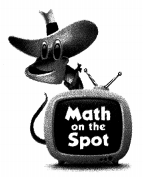It is given that
The crew on a fishing boat caught four fish that weighed a total of 1,092 pounds. The tarpon weighed twice as much as the amberjack and the white marlin weighed twice as much as the tarpon. The weight of the tuna was 5 times the weight of the amberjack.
Now,
Let the weight of the amberjack be: x Pounds
So,
The weight of the tarpon = 2x Pounds
The weight of the white marlin = 2 × 2x = 4x Pounds
The weight of the tuna = 5x Pounds
Now,
According to the given information,
x + 2x + 4x + 5x = 1,092 Pounds
12x = 1,092 Pounds
So,
x = $$\frac{1,092}{12}$$
Now,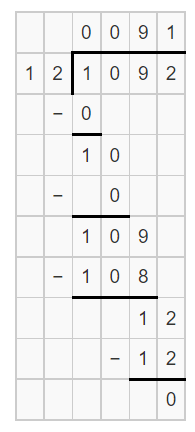So,
The weight of the amberjack is: 91 Pounds
The weight of the tarpon is: 182 Pounds
The weight of the white marlin is: 364 Pounds
The weight of the tuna is: 455 Pounds
Hence, from the above,
We can conclude that
The weight of the amberjack is: 91 Pounds
The weight of the tarpon is: 182 Pounds
The weight of the white marlin is: 364 Pounds
The weight of the tuna is: 455 Pounds

Question 4.
H.O.T. Multi-Step A fish market bought two swordfish at a rate of $13 per pound. The cost of the larger fish was 3 times as great as the cost of the smaller fish. The total cost of the two fish was$3,952. How much did each fish weigh?
It is given that
A fish market bought two swordfish at a rate of $13 per pound. The cost of the larger fish was 3 times as great as the cost of the smaller fish. The total cost of the two fish was$3,952
Now,
Let the cost of the smaller fish be: $x So, The cost of the larger fish is:$3x
Now,
The total cost of the two fishes per pound = ($3x +$x) × $13 =$52
Now,
According to the given information,
The weight of each fish = $3,952 ÷$52
Now,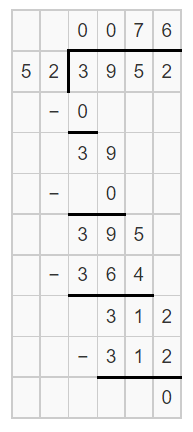So,
The weight of the smaller fish is: 76 Pounds
The weight of the larger fish is: 228 Pounds
Hence, from the above,
We can conclude that
The weight of the smaller fish is: 76 Pounds
The weight of the larger fish is: 228 Pounds

Question 5.
An ostrich at the zoo weighs 3 times as much as Mark’s pet dog. Together, the ostrich and the dog weigh 448 pounds. How much does the ostrich weigh?
(A) 149 pounds
(B) 112 pounds
(C) 224 pounds
(D) 336 pounds
It is given that
An ostrich at the zoo weighs 3 times as much as Mark’s pet dog. Together, the ostrich and the dog weigh 448 pounds
Now,
Let the weight of Mark’s pet dog be: x Pounds
So,
The weight of the ostrich is: 3x Pounds
Now,
According to the given information,
3x + x = 448 Pounds
4x = 448 Pounds
x = $$\frac{448}{4}$$
x = 112 Pounds
So,
The weight of the ostrich is: 336 Pounds
Hence, from the above,
We can conclude that
The weight of the ostrich at the zoo is:Question 6.
Use Diagrams A student drew this diagram to solve a word problem. Which statement matches what she drew?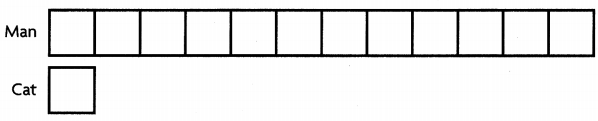(A) A man weighs 12 times as much as a cat.
(B) A cat weighs 12 times as much as a man.
(C) A man weighs 13 times as much as a cat.
(D) A cat weighs 13 times as much as a man.
It is given that
A student drew this diagram to solve a word problem
Now,
The given diagram is:Hence, from the above,
We can conclude that
The statement that matches with what the student drew is: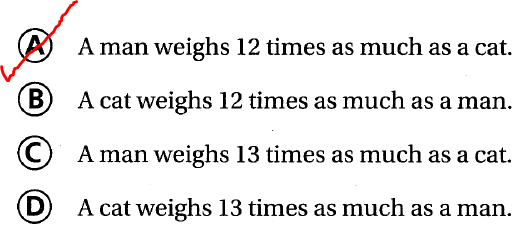Question 7.
Multi-Step A juice factory uses 4,650 pounds of pears and apples a day. The factory uses 30 times as many apples as pears. How many pounds of pears and how many pounds of apples does the factory use each day?
(A) 93 lb pears and 4,557 lb apples
(B) 150 lb pears and 4,500 lb apples
(C) 155 lb pears and 4,495 lb apples
(D) 310 lb pears and 4,340 lb apples
It is given that
A juice factory uses 4,650 pounds of pears and apples a day. The factory uses 30 times as many apples as pears
Now,
Let the weight of the pears be: x Pounds
So,
The weight of the apples is: 30x Pounds
Now,
According to the given information,
30x + x = 4,650
31x = 4,650
x = $$\frac{4,650}{31}$$
Now,So,
The weight of pears is: 150 Pounds
The weight of apples is: 4,500 Pounds
Hence, from the above,
We can conclude that
The number of pounds of pears and the number of pounds of apples does the factory use each day is:Texas Test Prep

Question 8.
Apply Captain James offers a deep-sea fishing tour. He charges $2,940 for a 14-hour trip. How much does each hour of the tour cost? (A)$201
(B) $138 (C)$210
(D) $294 Answer: It is given that Captain James offers a deep-sea fishing tour. He charges$2,940 for a 14-hour trip
Now,
According to the given information,
The cost of each hour of the tour = \$2,940 ÷ 14
Now,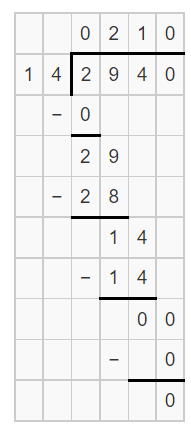Hence, from the above,
We can conclude that
The cost of each hour of the tour is:### Texas Go Math Grade 5 Lesson 2.8 Homework and Practice Answer Key

Eddie’s Moving Company moved 3 boxes out of Mrs. Diaz’s house. The box labeled “Kitchen” was 4 times heavier than the box labeled “Bedroom.” The box labeled “Library” was 14 times heavier than the box marked “Bedroom.” If the combined weight of the boxes was 817 pounds, how much did the box labeled “Kitchen” weigh?

Question 1.
What do I need to find?
I need to find the weight of the box labeled “Kitchen”

Question 2.
What information am I given?
I know that Eddie’s Moving Company moved 3 boxes out of Mrs. Diaz’s house. The box labeled “Kitchen” was 4 times heavier than the box labeled “Bedroom.” The box labeled “Library” was 14 times heavier than the box marked “Bedroom” and the combined weight of the boxes was 817 pounds

Question 3.
Draw a diagram.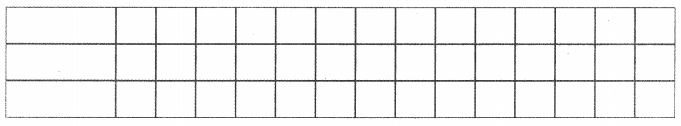The representation of the number of boxes in the form of a strip diagram is: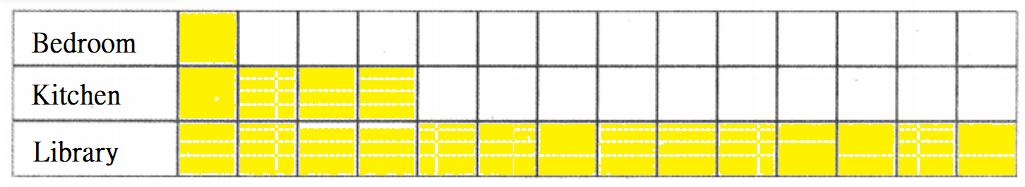Question 4.
Solve.
Let the weight of the box labeled “Bedroom” be: x Pounds
So,
x + 4x + 14x = 817 Pounds
19x = 817
So,
817 ÷ 19 = 43
So,
I can multiply 4 by 43 to find the weight of the “Kitchen” box.
So,
The box labeled “Kitchen” weighed 172 pounds.

Problem Solving

Question 5.
An athlete ran 1,200 laps around a track over a two-month period. He ran 4 times the number of laps in the second month than he ran in the first month. How many laps did the athlete run the first month?
It is given that
An athlete ran 1,200 laps around a track over a two-month period. He ran 4 times the number of laps in the second month than he ran in the first month
Now,
Let the number of laps run by an athlete in the first month be: x laps
So,
The number of laps run by an athlete in the first month be: 4x laps
Now,
According to the given information,
x + 4x = 1,200
5x = 1,200
x = $$\frac{1,200}{5}$$
x = 240 laps
Hence, from the above,
We can conclude that
The number of laps did the athlete run in the first month is: 240 laps

Question 6.
The diagram below compares the weights of a boy and a fish. Tell me what the diagram means.It is given that
The diagram below compares the weights of a boy and a fish
Now,
The given diagram is:Now,
From the given diagram,
We can observe that
The weight of the boy is 11 times as many as the weight of the fish
Hence, from the above,
We can conclude that
The given diagram represents:
The weight of the boy is 11 times as many as the weight of the fish

Lesson Check

Question 7.
Yani drew this diagram to solve a word problem. Which statement matches what she drew?(A) A hamster weighs 7 times as much as a duck.
(B) A duck weighs 7 times as much as a hamster.
(C) A hamster weighs 6 times as much as a duck.
(D) A duck weighs 6 times as much as a hamster.
It is given that
Yani drew this diagram to solve a word problem
Now,
The given diagram is:Hence, from the above,
We can conclude that
The statement that matches with what she drew is:Question 8.
An oak tree in Jake’s yard is 13 times taller than a model tree that he is making for a performance set. Together, the oak tree and model tree are 112 feet tall. How tall is the model tree?
(A) 8 feet
(B) 14 feet
(C) 26 feet
(D) 96 feet
It is given that
An oak tree in Jake’s yard is 13 times taller than a model tree that he is making for a performance set. Together, the oak tree and model tree are 112 feet tall
Now,
Let the height of the model oak tree be: x feet
So,
The height of the oak tree is: 13x feet
Now,
According to the given information,
13x + x = 112 feet
14x = 112 feet
x = $$\frac{112}{14}$$
x = 8 feet
Hence, from the above,
We can conclude that
The height of the model tree is:Question 9.
Lori’s book has 6 times more pages than Sal’s book. The combined number of pages for the two books is 532. How many pages does Lori’s book have?
(A) 76
(B) 88
(C) 456
(D) 532
It is given that
Lori’s book has 6 times more pages than Sal’s book. The combined number of pages for the two books is 532
Now,
Let the number of pages in Sai’s book be: x pages
So,
The number of pages in Lori’s book is: 6x pages
Now,
According to the given information,
x + 6x = 532
7x = 532
x = $$\frac{532}{7}$$
x = 76 pages
So,
The number of pages in Lori’s book = 76 × 6
= 456 pages
Hence, from the above,
We can conclude that
The number of pages in Lori’s book is:Question 10.
Raj drives his car 5 times farther than Gary drives his car. Together they drive 624 miles. How many miles does Rai drive?
(A) 520 miles
(B) 416 miles
(C) 104 miles
(D) 312miles
It is given that
Raj drives his car 5 times farther than Gary drives his car. Together they drive 624 miles
Now,
Let the distance driven by Gary be: x miles
So,
The distance driven by Raj is: 5x miles
Now,
According to the given information,
x + 5x = 624
6x = 624
x = $$\frac{624}{6}$$
x = 104 miles
So,
The distance driven by Raj is: 3,120 miles
Hence, from the above,
We can conclude that
The distance driven by Raj is: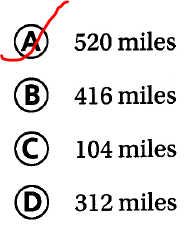Question 11.
Multi-Step The total number of books in the community’s mobile library is 1,155. There are 20 times more nonfiction books than fiction books. How many fiction books and how many nonfiction books are in the mobile library?
(A) 110 fiction and 1,045 nonfiction
(B) 55 fiction and 1,100 nonfiction
(C) 55 fiction and 1,045 nonfiction
(D) 1,100 fiction and 55 nonfiction
It is given that
The total number of books in the community’s mobile library is 1,155. There are 20 times more nonfiction books than fiction books
Now,
Let the number of fiction books be: x books
So,
The number of non-fiction books is: 20x books
Now,
According to the given information,
x + 20x = 1,155
21x = 1,155
x = $$\frac{1,155}{21}$$
x = 55 books
So,
The number of fiction books is: 55 books
The number of non-fiction books is: 1,100
Hence, from the above,
We can conclude that
The number of fiction books and nonfiction books that are in the mobile library is: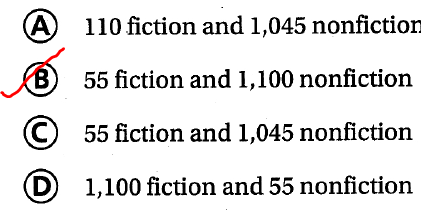Question 12.
Multi-Step Charlie’s weight is 5 times as great as the weight of his little sister, Tali. If the total weight of the two children is 132 pounds, how much does each child weigh?
(A) Tali: 22 pounds; Charlie: 110 pounds
(B) Tali: 110 pounds; Charlie: 22 pounds
(C) Tali: 44 pounds; Charlie: 88 pounds
(D) Tali: 88 pounds; Charlie: 44 pounds
It is given that
Charlie’s weight is 5 times as great as the weight of his little sister, Tali and the total weight of the two children is 132 pounds
Now,
Let the weight of Charlie’s little sister be: x Pounds
So,
The weight of Charlie is: 5x Pounds
Now,
According to the given information,
x + 5x = 132
6x = 132 Pounds
x = $$\frac{132}{6}$$
x = 22 Pounds
So,
The weight of Charlie’s little sister is: 22 Pounds
The weight of Charlie is: 110 Pounds
Hence, from the above,
We can conclude that
The weight of each child is:Scroll to Top